# Excel As CalculatorArticle byExcelMojo Team## What Is Excel As Calculator?

Excel as calculator enables one to quickly perform complex calculations and create appropriate formulas, Tables, and charts based on massive datasets. And we can calculate in a cell by entering the Equal To sign, followed by constants, cell references, and inbuilt functions, separated by arithmetic operators.

Users can use Excel as calculator to solve from simple arithmetic to complicated financial and statistical calculations.

For example, the table below shows two sets of values.

And the requirement is to find the product of the two values in each row and display the output in the corresponding target cell in column C.

Then, we can use the references to the cells containing the required values and the ‘*’ operator to find the product of the values in the corresponding target cells.

We enter the ‘=’ sign, the cell reference to the first value in column A, the ‘*’ operator, and the cell reference to the second value in column B in the target cell in each row of column C.

###### Key Takeaways
• Excel as calculator is an option to perform complicated calculations involving massive datasets quickly. While the program offers inbuilt functions, we can create customized formulas using constants, cell references, and inbuilt functions separated by arithmetic operators.
• Users can use Excel as a calculator to create complex formulas, Tables and plots, which will help perform financial and statistical calculations.
• We can enter the inbuilt functions directly in the required cells. Otherwise, we can apply the functions from the Formulas tab.
• We can use the Error Checking and Evaluate Formula options to troubleshoot errors due to the entered formulas.

### How To Use Excel As A Calculator?

The steps to use Excel as calculator are as follows:

1. Ensure the source dataset is valid and error-free.
2. Select a target cell where we must calculate using the source dataset values.
3. Enter the ‘=’ sign and the given data as a constant, cell reference, or Excel function containing the source data or references to the source data. Next, enter the applicable arithmetic operator such as ‘+’, ’‘, ‘*’, ‘/’, and ‘^’.

And then, enter the remaining required data, as mentioned previously, separated by the arithmetic operators.

• Press Enter to execute the formula and view the output in the target cell.

Furthermore, the following table shows the basic arithmetic operators and their Excel equivalent options. We can use them to create the appropriate formulas in a workbook to perform the required calculations.

### How To Calculate In Excel Sheet?

Let us see the steps to use Excel as calculator for calculating in a worksheet, with practical examples.

#### Example #1 – Use Formulas In Excel As A Calculator

We shall see an example of Excel as average calculator with formulas.

The table below shows the project points of students in three subjects.

And the requirement is to determine each student’s average project points based on the given data and display the output in column E.

Then, we can utilize Excel as average calculator by using the given data and the ‘+’ and ‘/’ operators in the target cells to achieve the required output.

Step 1: Select cell E3, enter the ‘=’ sign and the opening bracket, ‘(‘.

=(

Next, enter the first value, 9, followed by the ‘+’ operator.

Next, enter the second value, 8, followed by the ‘+’ operator.

And then, enter the third value, 9, closing bracket, ‘)’, and the Forward Slash, ‘/’.

Next, since we must calculate the average of three values, enter 3 as the denominator.

Step 2: Press Enter to obtain the required average project points of the first student.

Step 3: Select cell E4, enter the following formula, and press Enter.

=(10+10+10)/3

Likewise, select the remaining target cells one by one, enter the required formula, and press Enter to view the output for each student.

Thus, the above example shows we can use Excel as calculator for creating the required formulas using constants and arithmetic operators.

The formula in each target cell adds the three supplied project points. And then, it divides the sum by three to give the required average project points.

#### Example #2 – Use Cell References

We shall see an example of Excel as difference calculator using Excel cell references.

The table below shows each item’s maximum and minimum units, specified in column A, sold in January.

The requirement is to determine the difference between the maximum and minimum units of each item sold in January and display the output in column D.

Then, we can utilize Excel as difference calculator, using cell references and the ‘‘ sign in the target cells.

Step 1: Select cell D2, enter the ‘=’, the cell reference to the first required value specified in column B, and the ‘‘ sign.

=B2-

Next, enter the cell reference to the second required value specified in column C.

=B2-C2

Step 2: Press Enter to view the difference between the two referenced values in the target cell.

Step 3: Drag the Excel fill handle down towards the last target cell.

The formula will get applied to the remaining target cells.

Thus, the above example shows we can utilize Excel as calculator for creating the required formulas using Excel cell references and arithmetic operators.

The Excel subtraction formula in each target cell deducts the second value from the first to give the required difference.

#### Example #3 – Cell Reference Formulas Are Flexible

The following example shows how cell references make formulas flexible when using Excel as calculator.

The table below lists a firm’s employees and their date of birth data.

And consider we use the inbuilt Excel function YEAR, in column C to utilize Excel as year calculator. The function accepts a date as the argument value to determine the employees’ birth years.

So, we select the target cell and enter the ‘=’, the function name YEAR, ‘(‘, cell reference to the date value in the corresponding row, and ‘)’. And then, press Enter to obtain the required birth year.

And using the Excel fill handle, update the formula in the remaining target cells.

Next, assume the date of birth of the employee, Heather Walker is incorrect. And we must update the correct date of birth and year.

Then, the steps are as follows:

Step 1: Double-click at the end of the date value in cell B5 to place the cursor inside the specific cell and be in edit mode.

The date value will be in the default Excel date format.

So, press Backspace and enter the correct year.

And once we press Enter, cell B5 will show the updated date. And the formula output in cell C5 gets updated automatically.

Thus, the above Excel as year calculator example shows that when we use cell references in formulas, we do not have to update the formulas manually for changes in the input data.

#### Example #4 – Formula Cell Is Not Value, It Is The Only Formula

The following Excel as calculator example explains what happens when we copy a cell containing a formula and try pasting its content into another cell.

The table below shows the 2022-23 sales at a firm’s branch offices.

And the requirement is to determine the total sales in each year and display the output in cells B8:C9 using the Excel status bar and the addition formula.

Step 1: Select the cell range B2:B7 to view the total of the chosen values in the bottom-right corner in the status bar, \$213,000.

And enter the sum of the 2022 Sales values in cell B8.

Step 2: Select cell B9 and enter the following formula to add the 2022 Sales values.

=B2+B3+B4+B5+B6+B7

And press Enter to view the formula output in the target cell B9, \$213,000.

Step 3: Select cell B8 and use Ctrl + C to copy the cell data.

Next, choose cell C8 and use Ctrl + V to paste the copied data into the chosen cell.

We find the cell B8 value copied into cell C8 as the total 2023 Sales value.

Step 4: Choose cell B9 and use Ctrl + C to copy the cell data.

Next, select cell C9 and use Ctrl + V to paste the copied data into the chosen cell.

The above step copies the cell B9 formula into cell C9, with the cell references updated in the copied formula, and we obtain the correct total of 2023 Sales values in cell C9.

Thus, copying a formula containing cell references from a source cell into another applies the formula to the target cell instead of the source cell value.

#### Example #5 – Built-In Formulas Are Best Suited For Excel

We shall see an example of Excel as hours calculator to show that built-in functions help perform calculations quickly and error-free. And they are quite useful when the given dataset is massive and includes values in different formats.

For example, the table below lists tasks and their completion time details.

And the requirement is to determine the hour of completion of each task and display the output in column C.

Column B shows the task completion time data in unique formats. So, manually determining the required values will be an uphill task with a certain level of complexity.

Fortunately, Excel offers an inbuilt function, HOUR, to enable us to achieve the required output quickly when using Excel as hours calculator.

Step 1: Choose cell C2 and enter the HOUR().

=HOUR(

The HOUR() accepts the serial number equivalent to the time containing the required hour. And the input value can be a text string within double quotes, a decimal number, or other functions and formulas’ output.

Next, enter the cell reference to the required input value and close the bracket.

=HOUR(B2)

Step 2: Press Enter to view the HOUR() output in the target cell.

Furthermore, we can also enter the required inbuilt functions in a cell from the Function Library group in the Formulas tab.

Step 3: Using the fill handle, update the formula in the remaining target cells.

The cell C2 formula calculates 75% of 24 hours and returns the output 18, which is 6 pm.

Next, the cell C3 formula accepts an input containing a date and time. So, the function returns the hour part of the date and time value, 20, which is 8 pm.

Finally, the cell C4 formula input is a date. And as the time is not mentioned, Excel considers it 0 hours or 12 am. And hence, the function output in the specific target cell is 0.

### Important Things To Note

• When using Excel as calculator, start a formula in a cell with the ‘=’ sign.
• Using constants to create formulas and then copying the formulas into another cell will not automatically update the formula output in the target cell. Thus, it is best to use cell references in the formulas when copying them into other cells.
• Use Excel inbuilt functions, wherever possible, to perform the calculations in a worksheet quickly and accurately.

1. How to troubleshoot errors, such as #DIV/0! or #VALUE! when using Excel as calculator?

We can troubleshoot errors, such as #DIV/0! or #VALUE! when using Excel as calculator with the following options from the Formulas tab:

Trace Error
Evaluate Formula

For example, the following image shows two datasets.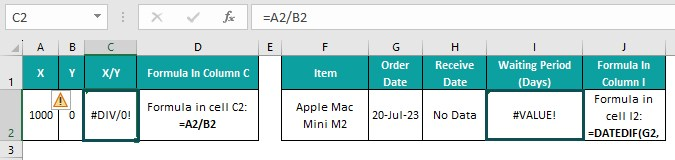We use ‘/’ to divide the cell A2 value by the cell B2 value in the target cell C2. And the formula results in the #DIV/0! error.

On the other hand, we use the DATEDIF() inbuilt function in the target cell I2 to determine the total number of days between the order and receive dates specified in cells G2 and H2. But the formula returns the #VALUE! error.

Here is how to troubleshoot the errors using the two methods mentioned above.

Step 1: Select cell C2. And then choose Formulas Error Checking drop-down – Error Checking.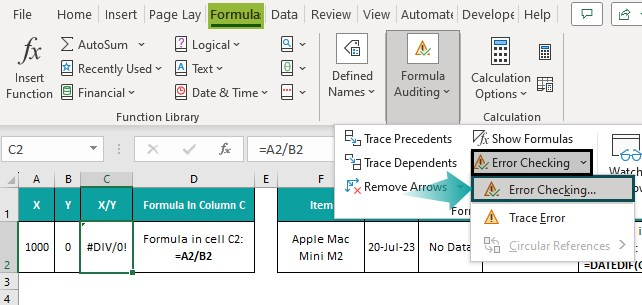The Error Checking window opens. And it shows the reason for the error.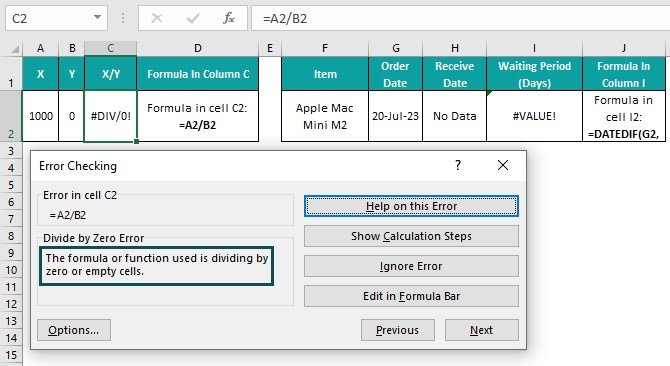Step 2: With cell C2 selected, click Formulas Error Checking drop-down – Trace Error.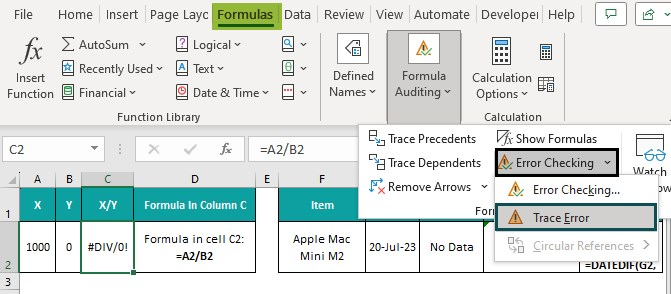Excel shows the values affecting the formula in the chosen cell using arrows.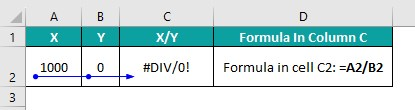And considering the reason cited in the Error Checking window, we find that the cell B2 value is 0, which is incorrect.

So, select cell B2 and enter the correct non-zero number to divide the cell A2 value.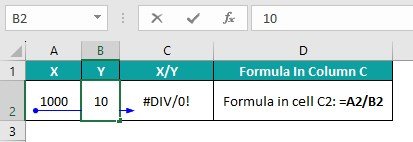And press Enter to view the correct output in the formula cell C2.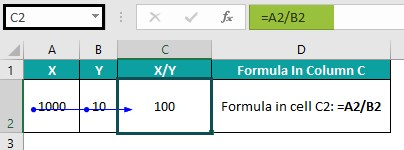Step 3: Select Formulas Remove Arrows.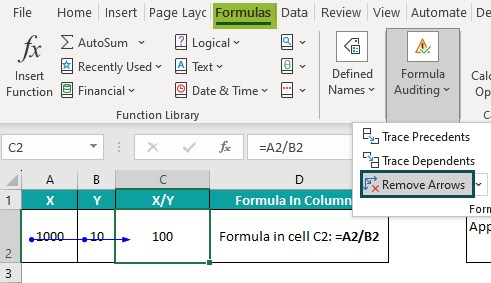The arrows get removed from the sheet.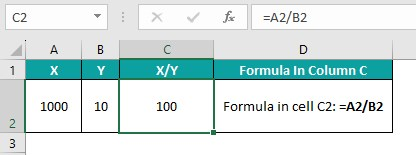Step 4: Select cell I2. And then, choose Formulas Error Checking drop-down – Error Checking.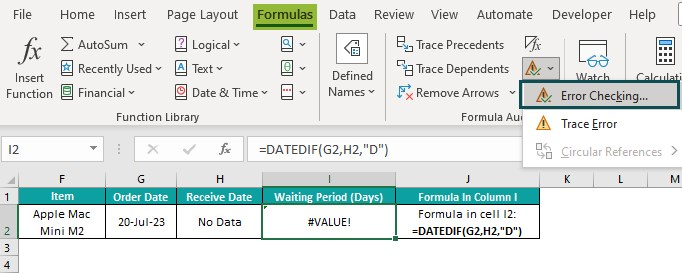The Error Checking window opens, where we can check the reason for the specific error.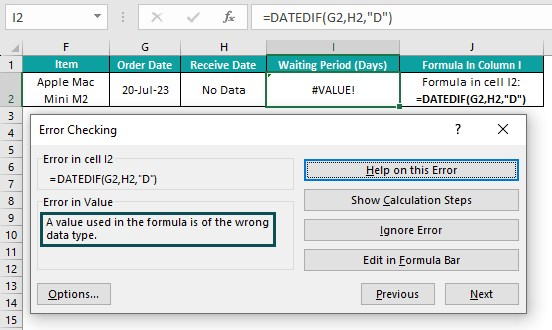Step 5: Choose cell I2 and Formulas Evaluate Formula.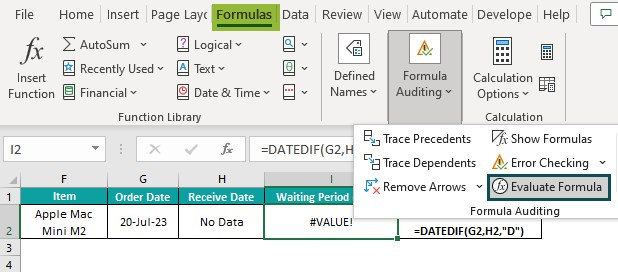The Evaluate Formula window opens, where we must click Evaluate to view the calculations step-by-step.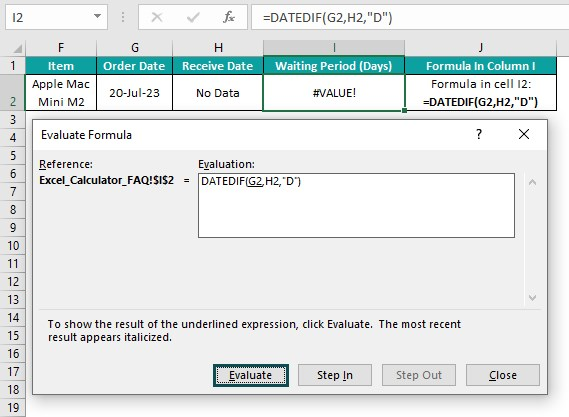Firstly, the first argument value, the cell reference G2, gets substituted by the corresponding value, the serial equivalent of the specified date, 45127. Click Evaluate.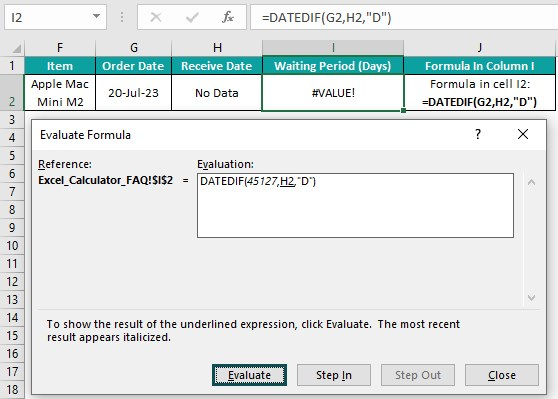Next, the second argument value, the cell reference H2, gets substituted by the corresponding value, “No Data”. Click Evaluate.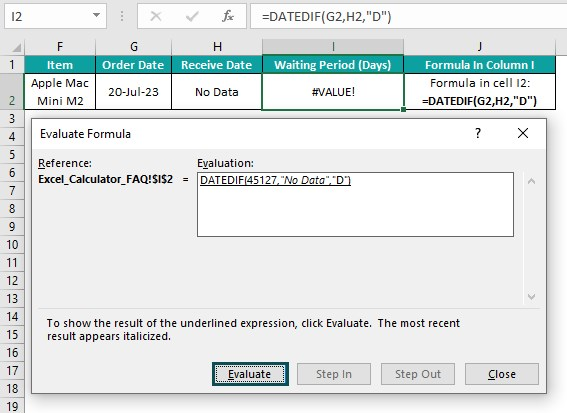And as the third argument is a value, the formula gets executed to return the error value. Click Close to exit from the Evaluate Formula window.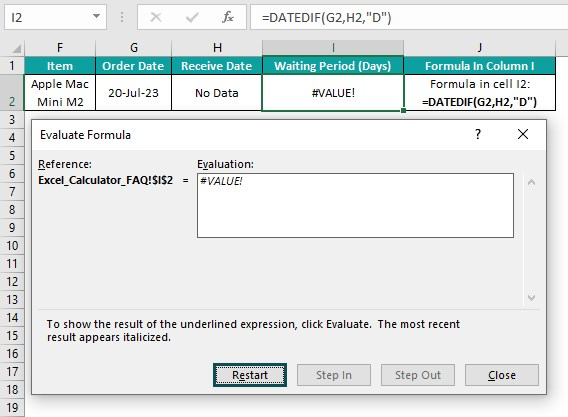Thus, considering the reason for the error mentioned in the Error Checking window and the evaluation steps, we can infer that the cell H2 value is causing the error.

So, we can select cell H2 and enter the required correct date.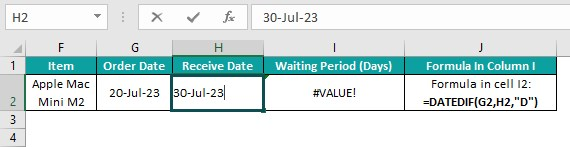Finally, pressing Enter will show the correct formula output instead of the error value in cell I2.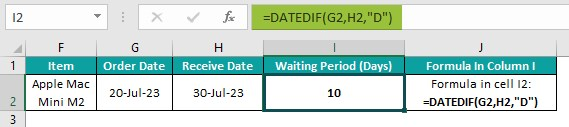2. Why is it that MS Excel is considered as calculator?

MS Excel is considered as calculator due to the following reasons:

We can quickly perform simple and complex mathematical calculations by creating formulas using constants, cell references, inbuilt functions and arithmetic operators.
Excel offers inbuilt functions that perform multiple steps of lengthy and tedious calculations and show the result at a click of the Enter key.
We can perform different calculations quickly, such as financial and statistical, involving massive datasets.
Excel allows us to create formulas, which we can copy into other cells to reuse to iterate the specific calculations in the required locations in a workbook.

3. Is Excel as calculator accurate?

Excel as calculator is accurate compared to other programs.
However, the accuracy may appear limited since its precision for any number is not more than 15 significant digits. And hence, we may face accuracy issues due to truncation, round-off, binary storage and cancellation at subtractions.